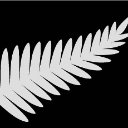Dear all,

am tring to use color grading shades  for the map I created but it's not showing any results. Am not sure if I have missing anything in my code. I will appreciate any help

```proc template;
parent=styles.listing;
style threecolorramp /startcolor=cream endcolor=darkblue;
end;
run;

proc gmap data=mydata map=mapsgfk.germany all;
id id;
choro cases / statistic=first discrete
levels=4
coutline=gray anno=myanno
cdefault=CXFFFFFF
legend=legend1;
run;
quit;
```
1 ACCEPTED SOLUTION

Accepted Solutions

Hmm ... I'm not sure if levels=4 and discrete can be used together(?)

Rather than "statistic=first discrete levels=4", try just "levels=4".

What does your data look like?

10 REPLIES 10ChrisNZ
Tourmaline | Level 20

you mean this?

yes I tried that but I get colors like red green yellow etc. Although they are not included

Try twocolorramp, rather than threecolorramp.

@GraphGuy This is the code I used but unfortunately no changes. Attached is my data

``````legend1 label =(position=top j=c h=10pt "Number of cases" )
value=(f="Arial" h=8pt c=black justify=left)
origin=(78,18)
across=1
position= (right middle)
offset=(0.5)
space=1
mode=reserve
shape= bar(.17in,.13in )/*width and height*/
;

proc template;
parent=styles.listing;
style twocolorramp /startcolor=cream endcolor=darkblue;
end;
run;

proc gmap data=mydata map=mydata all;
id id;
choro cases/ statistic=first discrete
levels=4
coutline=gray anno=myanno
cdefault=CXFFFFFF
legend=legend1;
run;
quit;``````

Hmm ... I'm not sure if levels=4 and discrete can be used together(?)

Rather than "statistic=first discrete levels=4", try just "levels=4".

What does your data look like?

@GraphGuy thanks it worked. I deleted discrete.

@GraphGuy  here is my data

the map data is mapsgfk.germany and  mapsgfk.germany_attr

Let's stick with one question per thread - this thread was for getting the gradient colors working, and we got that part working 🙂